Contents >> Engineering Mathematics >> Hydraulic Systems >> Dynamic Synthesis of Hydraulic Devices >> Examples of dynamic synthesis

 Dynamic synthesis of hydraulic devices - Examples Examples of dynamic synthesis of the throttle brake device of the hydro cylinder As examples of dynamic synthesis of throttle brake devices of hydro cylinders we’ll consider two simplified schemes: braking of the piston at action of impeding force and retraction of a rod (Fig. 2 а ) and at action of passing force at promotion of a rod (Fig. 3 а ). E x a m p l e 1. Let’s consider a variant of the simplified scheme (Fig. 2 а ). Input data for calculation: D =140 mm; d = 90 mm; L =1000 mm;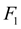= 90.32 cm²;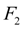=153.94 cm²;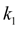= 12.64 cm²;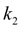=7.7 cm²;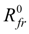= 3480 N; т = 46550 kg;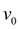=0.5 m/s; R =112.7 kN (impeding load);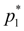= 31.4 М P а ;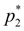= 31.4 М P а ;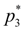= 0.735 М P а ;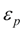= 3.92 М P а ;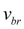= 0;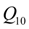= 271 l/min;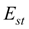= 980 МPа;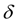=14 mm;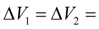25 cm³;= 1.96·10 5 МPа. S o l u t i o n.    For given values of parameters we’ll receive: from the formula (6)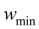= – 815.2 cm/s²; from the formula (16)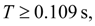let’s assume Т = 0.11s, then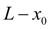= 3.3 cm;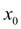= 96.7 cm. Coefficients of the polynomial (9) are equal: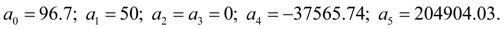Results of dynamic synthesis of the characteristic of the throttle brake device of the hydro cylinder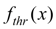for a considered simplified variant are resulted on Fig. 2 b . In the same place diagrams of change of pressures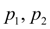and piston speed v are resulted at braking accordingly the planned law of movement.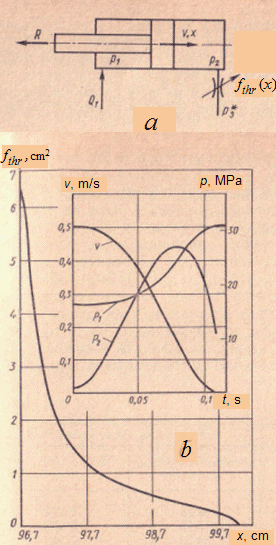Fig. 2. The simplified scheme ( a ) and results ( b ) of dynamic synthesis of the throttle brake device E x a m p l e  2. At synthesis of the characteristicof hydraulic damper for the second simplified case (Fig. 3 а ) the following input data have been accepted: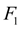=153.94  cm²;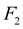= 90.32 cm²;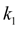= 7.7 cm²;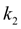=12.64 cm²; т = 63700 kg; R = –112.7 kN (passing force);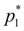= 0 (otherwise in view of differential of areas of the hydro cylinder for maintenance of the piston braking it is necessary to raise much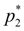);= 39.2 М P а ;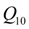=461.8 l/min; the rest parameters are as in the first simplified case. S o l u t i o n.    From the formula (6) it is found: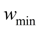= – 462.2 cm/s²; from the formula (16)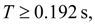let’s assume Т = 0.2 s, then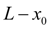= 6 cm;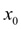= 94.0 cm. Coefficients of the polynomial (9) are equal: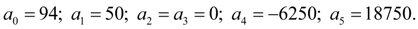Results of dynamic synthesis of the characteristic of the throttle brake device of the hydro cylinder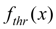for the second simplified variant are resulted on Fig. 3 b . In the same place diagrams of change of pressure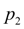and piston speed v are resulted at braking accordingly the planned law of movement.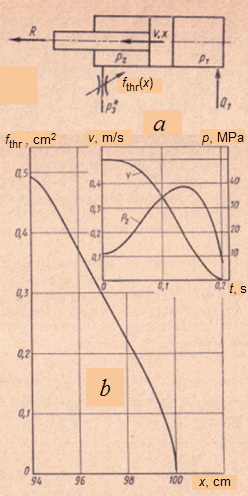Fig. 3. The simplified scheme ( a ) and results ( b ) of dynamic synthesis of the throttle brake device The characteristics of throttle brake devices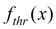received at certain parameters of the hydraulic system it is necessary then to survey at change of the reduced masses and loadings in all working range of the projected machine to define a degree of influence of each varied parameter to dynamics and basic parameters of braking process, otherwise, to define "scope" of the synthesized devices at change of external conditions.

 < Previous Contents Next >